Get inspired by the success stories of our students in IIT JAM MS, ISI  MStat, CMI MSc DS.  Learn More

# Conditions and Chance | ISI MStat 2018 PSB Problem 5This problem is a cute application of joint distribution and conditional probability. This is the problem 5 from ISI MStat 2018 PSB.

## Problem

Suppose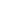and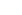are identically distributed random variables, not necessarily independent, taking values in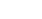. If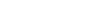and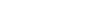obtain the joint distribution of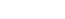.

## Solution

This problem is mainly about crunching the algebra of the conditions and get some good conditions for you to easily trail your path to the solution.

Usually, we go forward starting with the distribution ofandto the distribution of (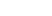). But, we will go backward from the distribution of () to,and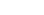with the help of conditional probability.

Now, observe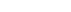becauseandare identically distributed.

Let's calculate the following:

•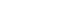andand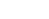•and•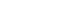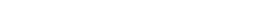####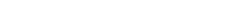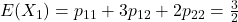Now,can take values {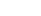}.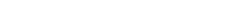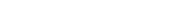.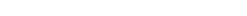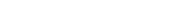.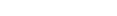or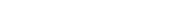.

####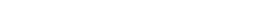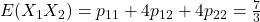.

Now, we need another condition, do you see that ?

####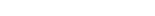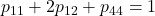.

Now, you can solve it easily to get the solutions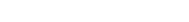.

### Food for Thought

Now, what do you think, how many expectation values will be required ifandtakes values in {1, 2, 3}?

What ifandtakes values in {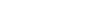}?

What if there are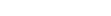taking values in {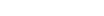}?

This is just another beautiful counting problem.

Enjoy and Stay Tuned!

This problem is a cute application of joint distribution and conditional probability. This is the problem 5 from ISI MStat 2018 PSB.

## Problem

Supposeandare identically distributed random variables, not necessarily independent, taking values in. Ifandobtain the joint distribution of.

## Solution

This problem is mainly about crunching the algebra of the conditions and get some good conditions for you to easily trail your path to the solution.

Usually, we go forward starting with the distribution ofandto the distribution of (). But, we will go backward from the distribution of () to,andwith the help of conditional probability.

Now, observebecauseandare identically distributed.

Let's calculate the following:

•andand•and•####Now,can take values {}...or.

####.

Now, we need another condition, do you see that ?

####.

Now, you can solve it easily to get the solutions.

### Food for Thought

Now, what do you think, how many expectation values will be required ifandtakes values in {1, 2, 3}?

What ifandtakes values in {}?

What if there aretaking values in {}?

This is just another beautiful counting problem.

Enjoy and Stay Tuned!

This site uses Akismet to reduce spam. Learn how your comment data is processed.

### Knowledge Partner